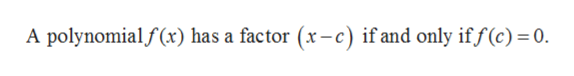# Practice Problem 1: Use the Factor Theorem to find zeros of f (x) = x3 + 4x2 -4x - 16 given that (x - 2) is a factor of the polynomial.

Question
3 viewshelp_outlineImage TranscriptionclosePractice Problem 1: Use the Factor Theorem to find zeros of f (x) = x3 + 4x2 - 4x - 16 given that (x - 2) is a factor of the polynomial. fullscreen
check_circle

Step 1

Given:

Step 2

Concept used:

Factor theorem: Suppose f(x) is a nonzero polynomial. Then (x-c) is a factor of f(x)  if and only if c is a zero of f(x).

That is,help_outlineImage TranscriptioncloseA polynomialf(x) has a factor (x-c) if and only if f (c) = 0. fullscreen
Step 3

From the given information (x-2) is a factor of polyno...

### Want to see the full answer?

See Solution

#### Want to see this answer and more?

Solutions are written by subject experts who are available 24/7. Questions are typically answered within 1 hour.*

See Solution
*Response times may vary by subject and question.
Tagged in